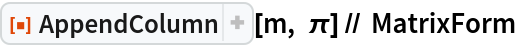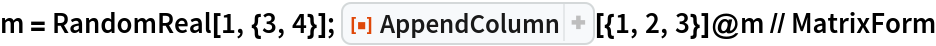Function Repository Resource:

# AppendColumn

Append a column to the right of a matrix

Contributed by: Michael Sollami
 ResourceFunction["AppendColumn"][matrix,list] appends a vector list as a column to matrix. ResourceFunction["AppendColumn"][matrix,s] appends a constant vector of scalar s to matrix. ResourceFunction["AppendColumn"][column] represents an operator form of AppendColumn that can be applied to a matrix.

## Details and Options

Dimensions of matrix and list must be compatible.

## Examples

### Basic Examples (2)

Append a column vector to a matrix:

 In:=Out=Append a scalar value to a matrix:

 In:=Out=### Scope (1)

Use AppendColumn as an operator form:

 In:=Out=Michael Sollami

## Version History

• 1.0.0 – 23 March 2020